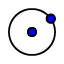# Exploring Rotation 1

Author:
Mr. Watson
Topic:
Rotation

## Question 1

Without moving the point of reflection, rotate the shape (using the angle of rotation slider) so that point A is at (-3,1). What angle did you rotate by? Was it a clockwise or counter clockwise rotation?

## Question 2

Without moving the point of reflection, rotate the shape (using the angle of rotation slider) so that point A is at (-1,-3). What angle did you rotate by? Now draw a circle using the circle with center through pointtool. First click on the center of rotation which is the point (0,0) (also called the origin), then click point A on the preimage (brown). Don't forget to change back to the selecttool before moving the slider.

As you rotate the figure (without moving the center of rotation) notice which points stay on the circle. Draw a new circle with center at the origin that passes through point B. Rotate the figure a bit. Do you see the pattern? Draw another circle with center at the origin and passing through a point on the preimage. Rotate the figure a bit. What is the pattern?

## Question 3

As you rotate the figure (without moving the center of rotation) notice which points stay on the circle. Draw a new circle so with center at the origin that passes through point B. rotate the figure a bit. Do you see the pattern? Draw another circle with center at the origin and passing through a point on the preimage. Rotate the figure a bit. What is the pattern?

Check your answers, redo any exercises that you missed, and then move on to Exploring Rotation 2.